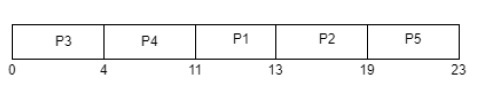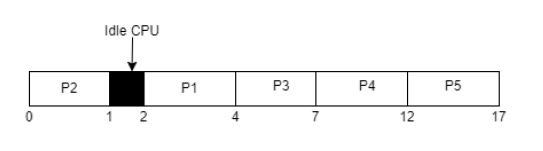# FCFS Scheduling

In case of multiprogramming, CPU needs to be scheduled, so that multiple works can be performed simultaneously in less time or at a same time. By CPU scheduling it is decided which of the processes in the ready queue is to be allocated in the CPU. Thus, there are so many CPU-scheduling algorithms in order to schedule CPU. FCFS scheduling is one of the CPU-scheduling algorithms.

## FCFS (FIRST-COME, FIRST-SERVED) Scheduling

FCFS is considered as simplest CPU-scheduling algorithm. In FCFS algorithm, the process that requests the CPU first is allocated in the CPU first. The implementation of FCFS algorithm is managed with FIFO (First in first out) queue. FCFS scheduling is non-preemptive. Nonpreemptive means, once the CPU has been allocated to a process, that process keeps the CPU until it executes a work or job or task and releases the CPU, either by requesting I/O.

## Real Life Example Of FCFS Scheduling

As a real life example of FCFS scheduling a billing counter system of shopping mall can be observed. The first person in the line gets the bill done first and then the next person gets the chance to get the bill and make payment and so on. If no priority is given to the VIP customers then the billing system will go on like this (means the first person (first task) in the line will get the bill first and after finishing (executing) the first customer’s payment the counter boy(CPU) will pay attention to other customers (separate tasks) as they are in the line). As FCFS is non-preemptive type so no priority will be given to the random important tasks.

## FCFS Scheduling Mathematical Examples

In CPU-scheduling problems some terms are used while solving the problems, so for conceptual purpose the terms are discussed as follows −

• Arrival time (AT) − Arrival time is the time at which the process arrives in ready queue.

• Burst time (BT) or CPU time of the process − Burst time is the unit of time in which a particular process completes its execution.

• Completion time (CT) − Completion time is the time at which the process has been terminated.

• Turn-around time (TAT) − The total time from arrival time to completion time is known as turn-around time. TAT can be written as,

Turn-around time (TAT) = Completion time (CT) – Arrival time (AT) or, TAT = Burst time (BT) + Waiting time (WT)

• Waiting time (WT) − Waiting time is the time at which the process waits for its allocation while the previous process is in the CPU for execution. WT is written as,

Waiting time (WT) = Turn-around time (TAT) – Burst time (BT)

• Response time (RT) − Response time is the time at which CPU has been allocated to a particular process first time.

In case of non-preemptive scheduling, generally Waiting time and Response time is same.

• Gantt chart − Gantt chart is a visualization which helps to scheduling and managing particular tasks in a project. It is used while solving scheduling problems, for a concept of how the processes are being allocated in different algorithms.

### Problem 1

Consider the given table below and find Completion time (CT), Turn-around time (TAT), Waiting time (WT), Response time (RT), Average Turn-around time and Average Waiting time.

Process ID

Arrival time

Burst time

P1

2

2

P2

5

6

P3

0

4

P4

0

7

P5

7

4

### Solution

Gantt chartFor this problem CT, TAT, WT, RT is shown in the given table −

Process ID

Arrival time

Burst time

CT

TAT=CT-AT

WT=TAT-BT

RT

P1

2

2

13

13-2= 11

11-2= 9

9

P2

5

6

19

19-5= 14

14-6= 8

8

P3

0

4

4

4-0= 4

4-4= 0

0

P4

0

7

11

11-0= 11

11-7= 4

4

P5

7

4

23

23-7= 16

16-4= 12

12

Average Waiting time = (9+8+0+4+12)/5 = 33/5 = 6.6 time unit (time unit can be considered as milliseconds)

Average Turn-around time = (11+14+4+11+16)/5 = 56/5 = 11.2 time unit (time unit can be considered as milliseconds)

### Problem 2

Consider the given table below and find Completion time (CT), Turn-around time (TAT), Waiting time (WT), Response time (RT), Average Turn-around time and Average Waiting time.

Process ID

Arrival time

Burst time

P1

2

2

P2

0

1

P3

2

3

P4

3

5

P5

4

5

### Solution

Gantt chart −For this problem CT, TAT, WT, RT is shown in the given table −

Process ID

Arrival time

Burst time

CT

TAT=CT-AT

WT=TAT-BT

RT

P1

2

2

4

4-2= 2

2-2= 0

0

P2

0

1

1

1-0= 1

1-1= 0

0

P3

2

3

7

7-2= 5

5-3= 2

2

P4

3

5

12

12-3= 9

9-5= 4

4

P5

4

5

17

17-4= 13

13-5= 8

8

Average Waiting time = (0+0+2+4+8)/5 = 14/5 = 2.8 time unit (time unit can be considered as milliseconds)

Average Turn-around time = (2+1+5+9+13)/5 = 30/5 = 6 time unit (time unit can be considered as milliseconds)

*In idle (not-active) CPU period, no process is scheduled to be terminated so in this time it remains void for a little time.

• It is an easy algorithm to implement since it does not include any complex way.

• Every task should be executed simultaneously as it follows FIFO queue.

• FCFS does not give priority to any random important tasks first so it’s a fair scheduling.

• FCFS results in convoy effect which means if a process with higher burst time comes first in the ready queue then the processes with lower burst time may get blocked and that processes with lower burst time may not be able to get the CPU if the higher burst time task takes time forever.

• If a process with long burst time comes in the line first then the other short burst time process have to wait for a long time, so it is not much good as time-sharing systems.

• Since it is non-preemptive, it does not release the CPU before it completes its task execution completely.

*Convoy effect and starvation sounds similar but there is slight difference, so it is advised to not treat these both terms as same words.

## Conclusion

Although FCFS is simple to implement and understand, FCFS is not good for interactive system and not used in modern operating systems. The Convoy effect in the FCFS can be prevented using other CPU-scheduling preemptive algorithms such as ‘Round-robin scheduling’.

Updated on: 05-Apr-2023

16K+ Views